Embedded Images Quiz I, Non Verbal Reasoning

# Embedded Images Quiz I, Non Verbal Reasoning - SSC CGL

Test Description

## 25 Questions MCQ Test Non Verbal Reasoning - Embedded Images Quiz I, Non Verbal Reasoning

Embedded Images Quiz I, Non Verbal Reasoning for SSC CGL 2023 is part of Non Verbal Reasoning preparation. The Embedded Images Quiz I, Non Verbal Reasoning questions and answers have been prepared according to the SSC CGL exam syllabus.The Embedded Images Quiz I, Non Verbal Reasoning MCQs are made for SSC CGL 2023 Exam. Find important definitions, questions, notes, meanings, examples, exercises, MCQs and online tests for Embedded Images Quiz I, Non Verbal Reasoning below.
Solutions of Embedded Images Quiz I, Non Verbal Reasoning questions in English are available as part of our Non Verbal Reasoning for SSC CGL & Embedded Images Quiz I, Non Verbal Reasoning solutions in Hindi for Non Verbal Reasoning course. Download more important topics, notes, lectures and mock test series for SSC CGL Exam by signing up for free. Attempt Embedded Images Quiz I, Non Verbal Reasoning | 25 questions in 50 minutes | Mock test for SSC CGL preparation | Free important questions MCQ to study Non Verbal Reasoning for SSC CGL Exam | Download free PDF with solutions
 1 Crore+ students have signed up on EduRev. Have you?
Embedded Images Quiz I, Non Verbal Reasoning - Question 1

### Directions to Solve In each of the following questions, you are given a figure (X) followed by four alternative figures (1), (2), (3) and (4) such that figure (X) is embedded in one of them. Trace out the alternative figure which contains fig. (X) as its part. Question - Find out the alternative figure which contains figure (X) as its part.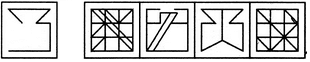(X)                (1)         (2)         (3)        (4)

Detailed Solution for Embedded Images Quiz I, Non Verbal Reasoning - Question 1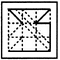Embedded Images Quiz I, Non Verbal Reasoning - Question 2

### Directions to Solve In each of the following questions, you are given a figure (X) followed by four alternative figures (1), (2), (3) and (4) such that figure (X) is embedded in one of them. Trace out the alternative figure which contains fig. (X) as its part. Question - Find out the alternative figure which contains figure (X) as its part.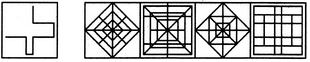(X)                (1)         (2)         (3)        (4)

Detailed Solution for Embedded Images Quiz I, Non Verbal Reasoning - Question 2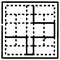Embedded Images Quiz I, Non Verbal Reasoning - Question 3

### Directions to Solve In each of the following questions, you are given a figure (X) followed by four alternative figures (1), (2), (3) and (4) such that figure (X) is embedded in one of them. Trace out the alternative figure which contains fig. (X) as its part. Question - Find out the alternative figure which contains figure (X) as its part.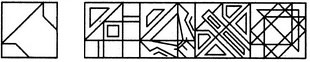(X)                (1)         (2)         (3)        (4)

Detailed Solution for Embedded Images Quiz I, Non Verbal Reasoning - Question 3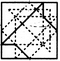Embedded Images Quiz I, Non Verbal Reasoning - Question 4

In each of the following questions, you are given a figure (X) followed by four alternative figures (1), (2), (3) and (4) such that figure (X) is embedded in one of them. Trace out the alternative figure which contains fig. (X) as its part.

Question -

Find out the alternative figure which contains figure (X) as its part.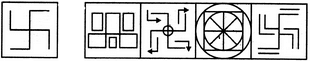(X)                (1)         (2)         (3)        (4)
Detailed Solution for Embedded Images Quiz I, Non Verbal Reasoning - Question 4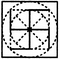Embedded Images Quiz I, Non Verbal Reasoning - Question 5

In each of the following questions, you are given a figure (X) followed by four alternative figures (1), (2), (3) and (4) such that figure (X) is embedded in one of them. Trace out the alternative figure which contains fig. (X) as its part.

Question -

Find out the alternative figure which contains figure (X) as its part.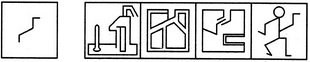(X)                (1)         (2)         (3)        (4)
Detailed Solution for Embedded Images Quiz I, Non Verbal Reasoning - Question 5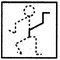Embedded Images Quiz I, Non Verbal Reasoning - Question 6

In each of the following questions, you are given a figure (X) followed by four alternative figures (1), (2), (3) and (4) such that figure (X) is embedded in one of them. Trace out the alternative figure which contains fig. (X) as its part.

Question -

Find out the alternative figure which contains figure (X) as its part.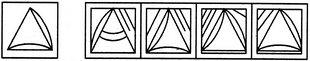(X)                (1)         (2)         (3)        (4)
Detailed Solution for Embedded Images Quiz I, Non Verbal Reasoning - Question 6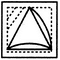Embedded Images Quiz I, Non Verbal Reasoning - Question 7

In each of the following questions, you are given a figure (X) followed by four alternative figures (1), (2), (3) and (4) such that figure (X) is embedded in one of them. Trace out the alternative figure which contains fig. (X) as its part.

Question -

Find out the alternative figure which contains figure (X) as its part.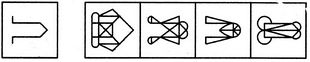(X)                (1)         (2)         (3)        (4)
Detailed Solution for Embedded Images Quiz I, Non Verbal Reasoning - Question 7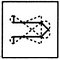Embedded Images Quiz I, Non Verbal Reasoning - Question 8

In each of the following questions, you are given a figure (X) followed by four alternative figures (1), (2), (3) and (4) such that figure (X) is embedded in one of them. Trace out the alternative figure which contains fig. (X) as its part.

Question -

Find out the alternative figure which contains figure (X) as its part.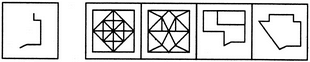(X)                (1)         (2)         (3)        (4)
Detailed Solution for Embedded Images Quiz I, Non Verbal Reasoning - Question 8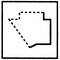Embedded Images Quiz I, Non Verbal Reasoning - Question 9

In each of the following questions, you are given a figure (X) followed by four alternative figures (1), (2), (3) and (4) such that figure (X) is embedded in one of them. Trace out the alternative figure which contains fig. (X) as its part.

Question -

Find out the alternative figure which contains figure (X) as its part.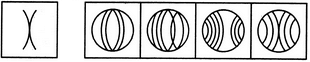(X)                (1)         (2)         (3)        (4)
Detailed Solution for Embedded Images Quiz I, Non Verbal Reasoning - Question 9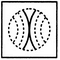Embedded Images Quiz I, Non Verbal Reasoning - Question 10

In each of the following questions, you are given a figure (X) followed by four alternative figures (1), (2), (3) and (4) such that figure (X) is embedded in one of them. Trace out the alternative figure which contains fig. (X) as its part.

Question -

Find out the alternative figure which contains figure (X) as its part.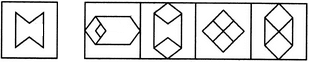(X)                (1)         (2)         (3)        (4)
Detailed Solution for Embedded Images Quiz I, Non Verbal Reasoning - Question 10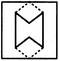Embedded Images Quiz I, Non Verbal Reasoning - Question 11

In each of the following questions, you are given a figure (X) followed by four alternative figures (1), (2), (3) and (4) such that figure (X) is embedded in one of them. Trace out the alternative figure which contains fig. (X) as its part.

Question -

Find out the alternative figure which contains figure (X) as its part.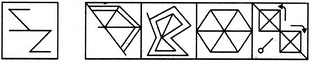(X)                (1)         (2)         (3)        (4)
Detailed Solution for Embedded Images Quiz I, Non Verbal Reasoning - Question 11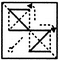Embedded Images Quiz I, Non Verbal Reasoning - Question 12

In each of the following questions, you are given a figure (X) followed by four alternative figures (1), (2), (3) and (4) such that figure (X) is embedded in one of them. Trace out the alternative figure which contains fig. (X) as its part.

Question -

Find out the alternative figure which contains figure (X) as its part.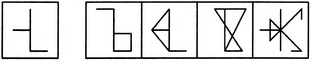(X)                (1)         (2)         (3)        (4)
Detailed Solution for Embedded Images Quiz I, Non Verbal Reasoning - Question 12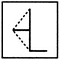Embedded Images Quiz I, Non Verbal Reasoning - Question 13

In each of the following questions, you are given a figure (X) followed by four alternative figures (1), (2), (3) and (4) such that figure (X) is embedded in one of them. Trace out the alternative figure which contains fig. (X) as its part.

Question -

Find out the alternative figure which contains figure (X) as its part.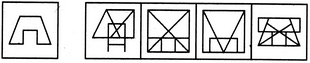(X)                (1)         (2)         (3)        (4)
Detailed Solution for Embedded Images Quiz I, Non Verbal Reasoning - Question 13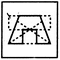Embedded Images Quiz I, Non Verbal Reasoning - Question 14

In each of the following questions, you are given a figure (X) followed by four alternative figures (1), (2), (3) and (4) such that figure (X) is embedded in one of them. Trace out the alternative figure which contains fig. (X) as its part.

Question -

Find out the alternative figure which contains figure (X) as its part.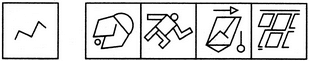(X)                (1)         (2)         (3)        (4)
Detailed Solution for Embedded Images Quiz I, Non Verbal Reasoning - Question 14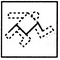Embedded Images Quiz I, Non Verbal Reasoning - Question 15

In each of the following questions, you are given a figure (X) followed by four alternative figures (1), (2), (3) and (4) such that figure (X) is embedded in one of them. Trace out the alternative figure which contains fig. (X) as its part.

Question -

Find out the alternative figure which contains figure (X) as its part.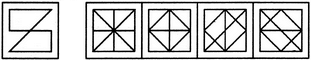(X)                (1)         (2)         (3)        (4)
Detailed Solution for Embedded Images Quiz I, Non Verbal Reasoning - Question 15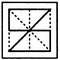Embedded Images Quiz I, Non Verbal Reasoning - Question 16

In each of the following questions, you are given a figure (X) followed by four alternative figures (1), (2), (3) and (4) such that figure (X) is embedded in one of them. Trace out the alternative figure which contains fig. (X) as its part.

Question -

Find out the alternative figure which contains figure (X) as its part.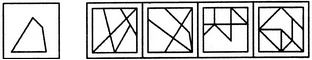(X)                (1)         (2)         (3)        (4)
Detailed Solution for Embedded Images Quiz I, Non Verbal Reasoning - Question 16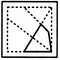Embedded Images Quiz I, Non Verbal Reasoning - Question 17

In each of the following questions, you are given a figure (X) followed by four alternative figures (1), (2), (3) and (4) such that figure (X) is embedded in one of them. Trace out the alternative figure which contains fig. (X) as its part.

Question -

Find out the alternative figure which contains figure (X) as its part.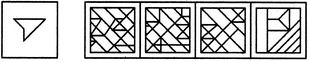(X)                (1)         (2)         (3)        (4)
Detailed Solution for Embedded Images Quiz I, Non Verbal Reasoning - Question 17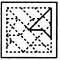Embedded Images Quiz I, Non Verbal Reasoning - Question 18

In each of the following questions, you are given a figure (X) followed by four alternative figures (1), (2), (3) and (4) such that figure (X) is embedded in one of them. Trace out the alternative figure which contains fig. (X) as its part.

Question -

Find out the alternative figure which contains figure (X) as its part.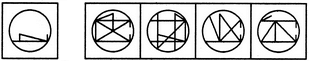(X)                (1)         (2)         (3)        (4)
Detailed Solution for Embedded Images Quiz I, Non Verbal Reasoning - Question 18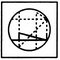Embedded Images Quiz I, Non Verbal Reasoning - Question 19

Directions to Solve

In each of the following questions, you are given a figure (X) followed by four alternative figures (1), (2), (3) and (4) such that figure (X) is embedded in one of them. Trace out the alternative figure which contains fig. (X) as its part.

Question -

Find out the alternative figure which contains figure (X) as its part.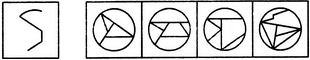(X)                (1)         (2)         (3)        (4)

Detailed Solution for Embedded Images Quiz I, Non Verbal Reasoning - Question 19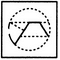Embedded Images Quiz I, Non Verbal Reasoning - Question 20

In each of the following questions, you are given a figure (X) followed by four alternative figures (1), (2), (3) and (4) such that figure (X) is embedded in one of them. Trace out the alternative figure which contains fig. (X) as its part.

Question -

Find out the alternative figure which contains figure (X) as its part.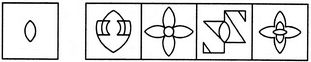(X)                (1)         (2)         (3)        (4)
Detailed Solution for Embedded Images Quiz I, Non Verbal Reasoning - Question 20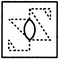Embedded Images Quiz I, Non Verbal Reasoning - Question 21

In each of the following questions, you are given a figure (X) followed by four alternative figures (1), (2), (3) and (4) such that figure (X) is embedded in one of them. Trace out the alternative figure which contains fig. (X) as its part.

Question -

Find out the alternative figure which contains figure (X) as its part.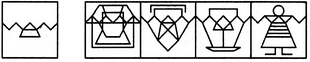(X)                (1)         (2)         (3)        (4)
Detailed Solution for Embedded Images Quiz I, Non Verbal Reasoning - Question 21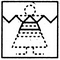Embedded Images Quiz I, Non Verbal Reasoning - Question 22

In each of the following questions, you are given a figure (X) followed by four alternative figures (1), (2), (3) and (4) such that figure (X) is embedded in one of them. Trace out the alternative figure which contains fig. (X) as its part.

Question -

Find out the alternative figure which contains figure (X) as its part.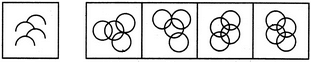(X)                (1)         (2)         (3)        (4)
Detailed Solution for Embedded Images Quiz I, Non Verbal Reasoning - Question 22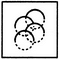Embedded Images Quiz I, Non Verbal Reasoning - Question 23

In each of the following questions, you are given a figure (X) followed by four alternative figures (1), (2), (3) and (4) such that figure (X) is embedded in one of them. Trace out the alternative figure which contains fig. (X) as its part.

Question -

Find out the alternative figure which contains figure (X) as its part.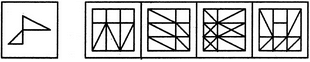(X)                (1)         (2)         (3)        (4)
Detailed Solution for Embedded Images Quiz I, Non Verbal Reasoning - Question 23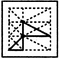Embedded Images Quiz I, Non Verbal Reasoning - Question 24

In each of the following questions, you are given a figure (X) followed by four alternative figures (1), (2), (3) and (4) such that figure (X) is embedded in one of them. Trace out the alternative figure which contains fig. (X) as its part.

Question -

Find out the alternative figure which contains figure (X) as its part.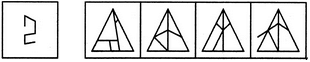(X)                (1)         (2)         (3)        (4)
Detailed Solution for Embedded Images Quiz I, Non Verbal Reasoning - Question 24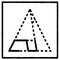Embedded Images Quiz I, Non Verbal Reasoning - Question 25

In each of the following questions, you are given a figure (X) followed by four alternative figures (1), (2), (3) and (4) such that figure (X) is embedded in one of them. Trace out the alternative figure which contains fig. (X) as its part.

Question -

Find out the alternative figure which contains figure (X) as its part.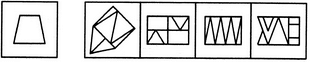(X)                (1)         (2)         (3)        (4)
Detailed Solution for Embedded Images Quiz I, Non Verbal Reasoning - Question 25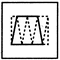## Non Verbal Reasoning

40 tests
Information about Embedded Images Quiz I, Non Verbal Reasoning Page
In this test you can find the Exam questions for Embedded Images Quiz I, Non Verbal Reasoning solved & explained in the simplest way possible. Besides giving Questions and answers for Embedded Images Quiz I, Non Verbal Reasoning, EduRev gives you an ample number of Online tests for practice

40 tests